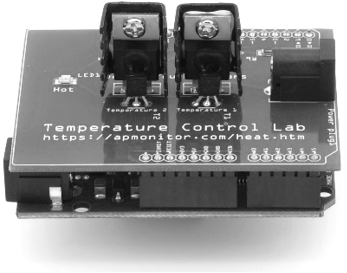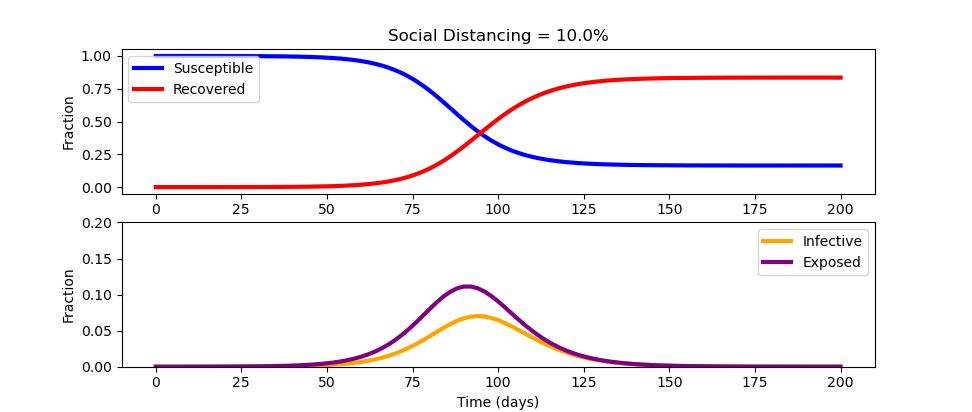## COVID-19 SEIR SimulationWith the spread of the disease COVID-19, epidemiologists have devised a strategy to "flatten the curve" by applying various levels of social distancing (sd). The strategy is to reduce the transmission of the virus SARS-CoV-2 so that the healthcare system is not overburdened.

Social distancing lowers the total number of people who are infected to keep hospitals below full capacity and reduces the total cumulative infected. Social distancing also minimizes the pandemic until a vaccine or effective treatments can be developed.

#### Economic Considerations

One of the downsides of extended social distancing is economic disruption where businesses fail, unemployment rises, supplies become scare, and assistance is needed to provide for the most vulnerable. Repeated outbreak cycles over multiple years are common when the virus mutates and the fraction of susceptible individuals is high. The objective of this exercise is to simulate the effect of social distancing to change the outcome over 200 days.

#### SEIR Compartmental Model

The fraction of Susceptible, Exposed, Infected, and Recovered (SEIR) population is given by a compartmental model with four differential equations.

$$\frac{ds}{dt} = -(1-u)\beta s i$$ $$\frac{de}{dt} = (1-u)\beta s i - \alpha e$$ $$\frac{di}{dt} = \alpha e - \gamma i$$ $$\frac{dr}{dt} = \gamma i$$

Susceptible (s): population fraction that is susceptible to the virus
Exposed (e): population fraction is infected with the virus but does not transmit to others
Infectious (i): population fraction that is infected and can infect others
Recovered (r): population fraction recovered from infection and is immune from further infection

This model is a simplification and neglects mortality and birth rates. There is also an opportunity to update the model with data sources such as search engine queries to improve early identification of regional outbreaks. Outbreak data on cruise ships give a controlled study to better assess under-reported cases. The model also neglects the variable fraction of infected patients that will need healthcare services. This fraction depends on how well vulnerable populations such as the elderly or immuno-compromised patients are protected.

Change the social distancing (u=0 (none), u=1 (total isolation)) to alter the outcome. A starting simulation model in Python predicts the response with a single social distancing factor (u=0) for 200 days for a campus population of 33,517.

u = 0.1  # social distancing (0-1)
# 0   = no social distancing
# 0.2 = masks and hybrid classes
# 0.3 = masks, hybrid, and online classes

Fill in the equations in the covid function definition. The first equation is dx = -(1-u)*beta * s * i.

dx = # dS/dt equation
dx = # dE/dt equation
dx = # dI/dt equation
dx = # dR/dt equation

Use the parameters for the SEIR model found in the Show Scipy ODEINT Starting Script.

import numpy as np
from scipy.integrate import odeint
import matplotlib.pyplot as plt

u = 0.1  # social distancing (0-1)
# 0   = no social distancing
# 0.2 = masks and hybrid classes
# 0.3 = masks, hybrid, and online classes

t_incubation = 5.1
t_infective = 3.3
R0 = 2.4
N = 33517 # students

# initial number of infected and recovered individuals
e0 = 1/N
i0 = 0.00
r0 = 0.00
s0 = 1 - e0 - i0 - r0
x0 = [s0,e0,i0,r0]

alpha = 1/t_incubation
gamma = 1/t_infective
beta = R0*gamma

def covid(x,t):
s,e,i,r = x
dx = np.zeros(4)
dx = # dS/dt equation
dx = # dE/dt equation
dx = # dI/dt equation
dx = # dR/dt equation
return dx

t = np.linspace(0, 200, 101)
x = odeint(covid,x0,t)
s = x[:,0]; e = x[:,1]; i = x[:,2]; r = x[:,3]

# plot the data
plt.figure(figsize=(8,5))

plt.subplot(2,1,1)
plt.title('Social Distancing = '+str(u*100)+'%')
plt.plot(t,s, color='blue', lw=3, label='Susceptible')
plt.plot(t,r, color='red',  lw=3, label='Recovered')
plt.ylabel('Fraction')
plt.legend()

plt.subplot(2,1,2)
plt.plot(t,i, color='orange', lw=3, label='Infective')
plt.plot(t,e, color='purple', lw=3, label='Exposed')
plt.ylim(0, 0.2)
plt.xlabel('Time (days)')
plt.ylabel('Fraction')
plt.legend()

plt.show()

#### Solution

The source code for the simulation solution is shown below. Select the ODEINT or Gekko solutions to see the source code only after you have completed the assignment and need to check your solution. Also, there is the Python Notebook on Modeling and Control of a Campus COVID-19 Outbreak by Jeff Kantor at Notre Dame.import numpy as np
from scipy.integrate import odeint
import matplotlib.pyplot as plt

u = 0.1  # social distancing (0-1)
# 0   = no social distancing
# 0.2 = masks and hybrid classes
# 0.3 = masks, hybrid, and online classes

t_incubation = 5.1
t_infective = 3.3
R0 = 2.4
N = 33517 # students

# initial number of infected and recovered individuals
e0 = 1/N
i0 = 0.00
r0 = 0.00
s0 = 1 - e0 - i0 - r0
x0 = [s0,e0,i0,r0]

alpha = 1/t_incubation
gamma = 1/t_infective
beta = R0*gamma

def covid(x,t):
s,e,i,r = x
dx = np.zeros(4)
dx = -(1-u)*beta * s * i
dx = (1-u)*beta * s * i - alpha * e
dx = alpha * e - gamma * i
dx = gamma*i
return dx

t = np.linspace(0, 200, 101)
x = odeint(covid,x0,t)
s = x[:,0]; e = x[:,1]; i = x[:,2]; r = x[:,3]

# plot the data
plt.figure(figsize=(8,5))

plt.subplot(2,1,1)
plt.title('Social Distancing = '+str(u*100)+'%')
plt.plot(t,s, color='blue', lw=3, label='Susceptible')
plt.plot(t,r, color='red',  lw=3, label='Recovered')
plt.ylabel('Fraction')
plt.legend()

plt.subplot(2,1,2)
plt.plot(t,i, color='orange', lw=3, label='Infective')
plt.plot(t,e, color='purple', lw=3, label='Exposed')
plt.ylim(0, 0.2)
plt.xlabel('Time (days)')
plt.ylabel('Fraction')
plt.legend()

plt.show()import numpy as np
from gekko import GEKKO
import matplotlib.pyplot as plt

m = GEKKO()
u = m.FV(0.1)   # social distancing (0-1)
# 0   = no social distancing
# 0.2 = masks and hybrid classes
# 0.3 = masks, hybrid, and online classes

t_incubation = 5.1
t_infective = 3.3
R0 = 2.4
N = 33517

# initial number of infected and recovered individuals
e_initial = 1/N
i_initial = 0.00
r_initial = 0.00
s_initial = 1 - e_initial - i_initial - r_initial

alpha = 1/t_incubation
gamma = 1/t_infective
beta = R0*gamma

s,e,i,r = m.Array(m.Var,4)
s.value = s_initial
e.value = e_initial
i.value = i_initial
s.value = s_initial
m.Equations([s.dt()==-(1-u)*beta * s * i,\
e.dt()== (1-u)*beta * s * i - alpha * e,\
i.dt()==alpha * e - gamma * i,\
r.dt()==gamma*i])

t = np.linspace(0, 200, 101)
t = np.insert(t,1,[0.001,0.002,0.004,0.008,0.02,0.04,0.08,\
0.2,0.4,0.8])
m.time = t
m.options.IMODE=7
m.options.NODES=3
m.solve(disp=False)

# plot the data
plt.figure(figsize=(8,5))
plt.subplot(2,1,1)
plt.title('Social Distancing = '+str(u.value*100)+'%')
plt.plot(m.time, s.value, color='blue', lw=3, label='Susceptible')
plt.plot(m.time, r.value, color='red',  lw=3, label='Recovered')
plt.ylabel('Fraction')
plt.legend()

plt.subplot(2,1,2)
plt.plot(m.time, i.value, color='orange', lw=3, label='Infective')
plt.plot(m.time, e.value, color='purple', lw=3, label='Exposed')
plt.ylim(0, 0.2)
plt.xlabel('Time (days)')
plt.ylabel('Fraction')
plt.legend()

plt.show()

#### Further Reading: Optimize the Curve

When a constant social distancing policy is enforced, the outbreak peaks before receding. Over-aggressive social distancing also creates economic damage. There are optimization methods to create an optimal distancing policy that changes over time to not only flatten the curve but also optimize to available healthcare constraints. Optimization is covered in courses such as Design Optimization and Machine Learning and Dynamic Optimization.

#### References

• Kantor, J., Modeling and Control of a Campus COVID-19 Outbreak, 2020, Video Link.
• Keeling, Matt J., and Pejman Rohani. Modeling Infectious Diseases in Humans and Animals. Princeton University Press, 2008. JSTOR, www.jstor.org/stable/j.ctvcm4gk0. Accessed 25 Feb. 2020.
• Pan, Jinhua, et al. Effectiveness of control strategies for Coronavirus Disease 2019: a SEIR dynamic modeling study. medRxiv (2020).
• Peng, Liangrong, et al. Epidemic analysis of COVID-19 in China by dynamical modeling. arXiv preprint arXiv:2002.06563 (2020).
• Cook, S., et al., Assessing Google flu trends performance in the United States during the 2009 influenza virus A (H1N1) pandemic, PloS one 6(8): e23610, 2011.
• Ginsberg, J., et al., Detecting influenza epidemics using search engine query data, Nature 457.7232: 1012-1014, 2009.
• Word, D.P., Abbott, G.H., Cummings, D., Laird, C.D., Estimating seasonal drivers in childhood infectious diseases with continuous time and discrete-time models, Proc. 2010 American Control Conference, Baltimore, MD, 5137-5142, June 2010.
• Beal, L.D.R., Hill, D., Martin, R.A., and Hedengren, J. D., GEKKO Optimization Suite, Processes, Volume 6, Number 8, 2018, doi: 10.3390/pr6080106.
• Hedengren, J.D., Asgharzadeh Shishavan, R., Powell, K.M., Edgar, T.F., Nonlinear Modeling, Estimation and Predictive Control in APMonitor, Computers and Chemical Engineering, 70, 133–148, doi:10.1016/j.compchemeng.2014.04.013, 2014.
• Lewis, N.R., Hedengren, J.D., Haseltine, E.L., Hybrid Dynamic Optimization Methods for Systems Biology with Efficient Sensitivities, Processes 3:3, 701-729, doi:10.3390/pr3030701, 2015.
• Carneiro, H.A., Mylonakis, E., Google trends: a web-based tool for real-time surveillance of disease outbreaks, Clinical infectious diseases 49.10: 1557-1564, 2009.
• Vishal S. Arora, Martin McKee, David Stuckler, Google Trends: Opportunities and limitations in health and health policy research, Health Policy, 123: 3, 2019, 338-341, 2019.
• Abbott, C.S., Haseltine, E.L., Martin, R.A., Hedengren, J.D., New Capabilities for Large-Scale Models in Computational Biology, Computing and Systems Technology Division, AIChE National Meeting, Pittsburgh, PA, Oct 2012.
• Polwiang, S., The seasonal reproduction number of dengue fever: impacts of climate on transmission, PeerJ 3:e1069, doi:10.7717/peerj.1069, 2015.
• Word, D.P., et al. Interior-point methods for estimating seasonal parameters in discrete-time infectious disease models, PloS one 8.10: e74208, 2013.